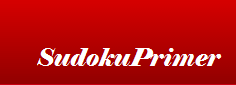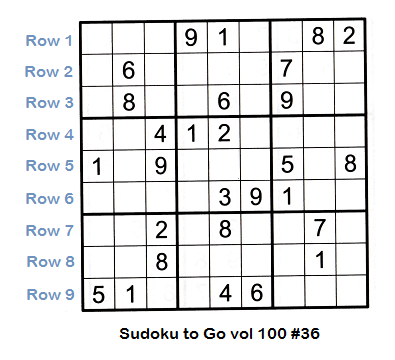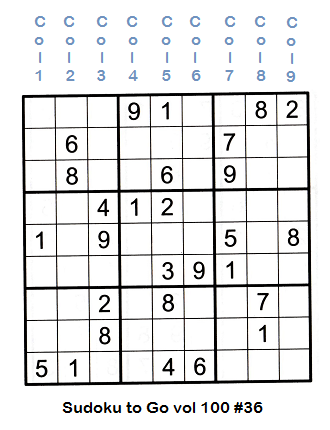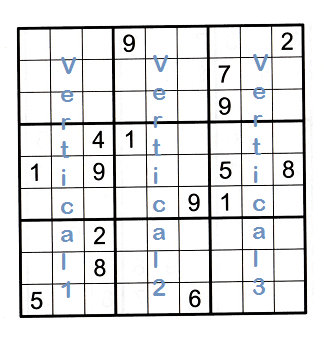﻿
Sudoku Primer & Complete Reference# Sudoku Glossary of Terms

Understanding the sudoku terminology on this page will help you both on this website and in other places that discuss sudoku. A quick review of these sudoku concepts will help you understand what is meant as you run across the differnet terms.

Use this page to become familiar with the terms on this site. You can refer to it when you see a term you don't understand. It is recommended that you at least skim through the list of terms and read the ones you aren't familiar with so you recognize them when you see them.

Box
A box is a series of nine cells organized in three mini-rows and three mini-columns within the box. The sudoku grid has 81 cells with nine overlaid boxes (three wide and three high). Boxes are numbered from the top left (box 1) to the bottom right (box 9). See container numbering below for more information. Boxes are one of the three container types. See also container.

Cell
A cell is the smallest unit in a Sudoku puzzle. A cell is a box that contains one number. There are 81 cells in a sudoku grid.

Column
A column is a vertical series of nine adjacent cells. Each column holds the numbers 1 through 9. Every number exists in each column and no number repeats. Columns are one of the three container types. See containers.

Container
Containers are groups of cells that contain an entire set of nine numbers. There are three types of containers - rows, columns and boxes. See rows, columns and boxes on this page.

Container numbering
The Sudoku grid containers are numbered to aid in illustration when explaining concepts. The three containers (see container on this page) are each numbered 1-9. The illustrations below show how each container is numbered. This should be very straightforward and easy to remember.

Rows are numbered 1 through 9 from the top row to the bottom row.Columns are numbered 1 through 9 from the left column to the right column.Boxes are numbered 1 through 9 from the top left to the bottom right.Givens
All standard sudoku puzzles start out with numbers that are already filled in by the puzzle creator. These numbers are called givens.

Sudoku puzzles have nine rows and nine columns which gives 91 total numbers. Research shows that a puzzle must have at least 17 givens. If it has less than 17 givens there will be more than one solution.

Easier puzzles generally have more givens and harder puzzles have fewer. However the skill level of a puzzle is not entirely determined by the number of givens.

Notation of cells, rows, columns, boxes and sections
When people communicate about sudoku they need a way to describe it in text form. When a cell is specified, or when certain combinations of cells are described, a shortcut notation is used to specify its location. This shortcut notation is explained here.

What does r2c3 mean?
A single cell is called out by specifying its row and column numbers with an 'r' preceding the row number and a 'c' preceding the column number. Examples: r1c9 means the cell in row one and column nine (the rightmost cell on the top row). r8c3 is the cell on row eight, third cell from the left. Hopefully this is straight-forward and easy to understand.

Rows are specified by their number and columns are called out by their number. Row one is the top row, row nine is the bottom row. Column one is the leftmost column, column nine is the rightmost column. boxes use the same numbering as rows and columns. box one is the top left box. box five is the very middle box, and box nine is the bottom right box.

What is vs2?
Sections are specified using either horizontal or vertical and a number (1, 2 or 3). Examples: the topmost section is hs1. The rightmost section is vs3.

How about 23t or 345t?
Twin and triplet notation is similar to row/column notation. Twins are shown using the two numbers that are twins with a 't' behind them. For example 23t means twins that are 2 and 3. Note that there are two digits preceding the 't' when they are twins.

When there are three digits before the 't' a set of triplets is described. For example 345t means a container has triplets and they are the numbers 3, 4 and 5.

Patterns
A pattern is a combination of filled and unfilled cells in a box. Patterns are not fundamental to solving Sudoku but they can help you solve them.

In the future sudokuPrimer will have a whole series of patterns that can help you solve puzzles. You will learn how to use each pattern. Check back for patterns, hopefully coming soon.

Row
A row is a horizontal series of nine adjacent cells. Each row holds the numbers 1 through 9. Every number exists in each row and no number repeats. Rows are one of the three container types. See containers.

Section
A section is a combination of three rows or columns, and three boxes. A section is not one of the basic building blocks of a Sudoku puzzle. However sections are useful in solving them. Three boxes across or three boxes down comprise a section.

To help illustrate sections think of three boxes in a line, either horizontally or vertically. There are three horizontal sections.• boxes one, two and three comprise the top horizontal section
• boxes four, five and six make up the middle horizontal section
• boxes seven, eight and nine are the three boxes in the bottom horizontal section
There are also three vertical sections.• boxes one, four and seven make up the left vertical section
• boxes two, five and eight together make up the center vertical section
• boxes three, six and nine comprise the rightmost vertical section
See "Solving sections" in techniques to learn how to solve them.